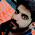## Saturday, September 19, 2009

### Activity related to Pythagoras Theorem

This project is contributed by Mayank X-B
Aim-To prove Pythagoras theorem by paper cutting and pasting.
Material Required-Thermocol,coloured sheets,cutter,scissors,sketchpens
ruler,fevistick .
Procedure-1 On a coloured sheet of paper draw triangle ABC with AB=3
units, BC=4 units and AC=5 units. Cut it out. (Taking 1 unit=1.5 inches)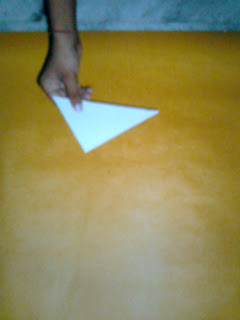2 Paste this triangle on a coloured sheet of paper covering a
thermocol

.3 On AB paste a square ABDE of side=3 units

.4 On BC paste a square BCFG of side=4 units.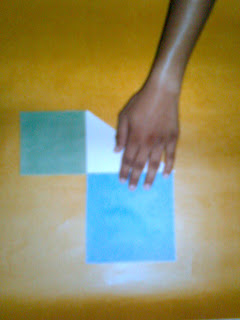5 On AC paste a square ACHI of side=5 units.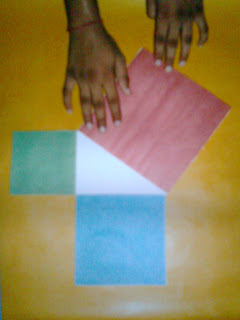6 Make replicas of squares ABDE and BCFG.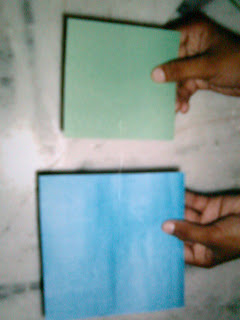7 Cut the replica of ABDE into 9 small squares each of area=1 sq unit.8 Cut the replica of BCFG into 16 small squares each of area=1 sq unit.9 Paste the unit squares obtained in step-7 and step-8 on square
ACHI.Observation-The unit squares overlap square ACHI completely.Result-Therefore, area of square ABDE + area of square BCFG = area of square
ACHI , ie ,
AB^2+ BC^2 = AC^2

1.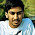2.